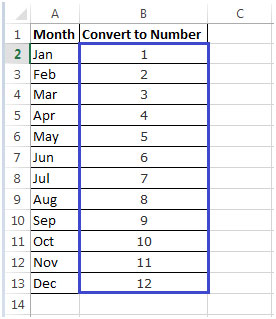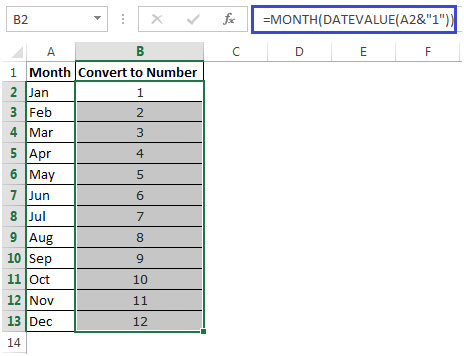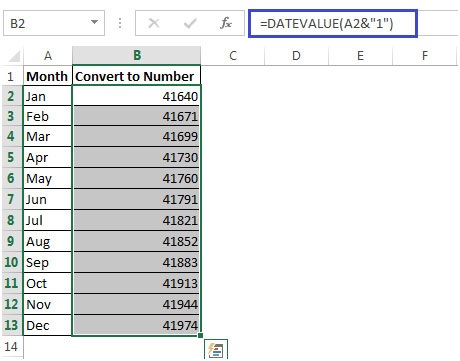# Converting Month Name to a Number in Microsoft Excel

In this article, we will learn how to convert the month name to a number in Microsoft Excel 2010.

While preparing reports there could be a requirement to convert a column that holds the name of the month (January, February, etc) into a number (1, 2).

Let us understand with an example:

• Column A contains Months Jan, Feb, Mar, till Dec.
• We want to convert the Months to a Number. i.e. Jan=1, Feb =2 & so on as shown below which has been input manually.We can use a combination of MONTH & DATEVALUE functions to get result.

• We can use the following formula in cell B2& then copy down to B3:B13 as shown in the below picture to get the output
• In cell B2=MONTH(DATEVALUE(A2&"1"))• The DateValue formula will convert the Month say Jan to 41640. If we format the number 41640 to Date Format, it will return 1/1/2014• Enclosing the Date value formula for the Month Formula will convert the number 41640 to 1.
• Thus, we get the required output.In this way, we can convert the name of the month to the number of the month.

Popular Articles:

50 Excel Shortcuts to Increase Your Productivity

How to use the VLOOKUP Function in Excel

How to use the COUNTIF function in Excel

How to use the SUMIF Function in Excel

1.2.